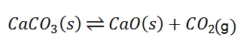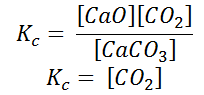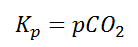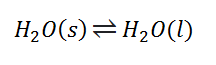Checkout JEE MAINS 2022 Question Paper Analysis : Checkout JEE MAINS 2022 Question Paper Analysis :

# Heterogeneous Equilibrium

## Introduction

When the state of equilibrium in a system has components in more than one phase it is termed as a heterogeneous equilibrium. For example, if we take a container with ice and water at a temperature that is allowing the existence of both the phases simultaneously, both ice and water are present in a state of equilibrium. This state is termed as heterogeneous equilibrium.

## Equilibrium in heterogeneous System

In a chemical reaction the reacting species combine with each other to form products. If such a reaction is carried in a closed container, in many cases, we find that it is not completed and after some time , the reaction mixture contains both the reactant and product species. Moreover, the concentration of these species also becomes constant after some time , provided both the temperature and the pressure are constant . Under the conditions, the reaction is said to be equilibrium.

In a heterogeneous system involving a reversible reaction, the reactants and products are in the different phases.

Let us illustrate the following reversible reaction involving decomposition of CaCO3 (s). Calcium carbonate on decomposition to produce CaO and CO2 . At a time the rate at which CaCO3 (s) decomposes is the same at the product combine to give the reactant. At this time equilibrium takes place.## Examples of heterogeneous Equilibrium

The examples of a heterogeneous equilibrium are

• Reaction of solid Ferrous oxide with Gaseous carbon monoxide produces solid iron and gaseous carbon dioxide.

FeO (s) + CO (g) ⇌ Fe (s) + CO2(g)

• Reaction of steam with red hot carbon produces hydrogen gas and carbon monoxide gas.

H2O (g) + C (s) ⇌ H2(g) + CO(g)

• Reaction of carbon dioxide gas with solid carbon at equilibrium produces carbon monoxide gas.

CO2 (g) + C (s) ⇌ 2 CO(g)

## Equilibrium constant in heterogeneous System

Writing the equilibrium constant for heterogeneous reactions is different from that of the homogeneous reactions. For example, consider the thermal dissociation of calcium carbonate into calcium oxide and carbon dioxide. Here, we can see that the equilibrium constant for the dissociation of the reactant into its products is only dependent on the gaseous component, as the solid and the liquid reactants are considered to be constant.Here, the components CaCO3 and CaO are solids, so their molar concentration remains constant throughout the reaction. Therefore, the equilibrium constant can be written as,Also, in terms of Kp, we can writeWhere p denotes the partial pressure. In other words, we can state that, at a given temperature, there is a constant concentration or partial pressure of CO2 in the equilibrium reaction with CaO and CaCO3.

Similarly: for equation CO2 (g) + C (s) ⇌ 2 CO(g)

Equilibrium constant Kc = [ CO]2 / [CO2]

## Equilibrium in Physical change:

The phase changes are also heterogeneous equilibrium systems. We know that solid , liquid and gas are the state of substance so that types of equilibrium are possible between

Solid – Liquid Equilibrium (solid ⇋ liquid)

Liquid – Gas Equilibrium (liquid ⇋ Gas)

Solid – Gas Equilibrium (solid ⇋ Gas)

1. Solid – Liquid Equilibrium:

The equilibrium between ice and water at 0℃ and 1 atm pressure in a thermo flask . In this case the rate of melting of ice and freezing water are taking place at the same rate.

In terms of its equation, this can be written as:1. Liquid – Gas Equilibrium:

The liquid -gas equilibrium can be illustrated with the help of evaporation of water in a closed container at room temperature.

H2O (l) ⇋ H2O (g)

1. Solid – Gas Equilibrium:

This state of equilibrium takes place in case of substances like ammonium chloride, iodine, camphor etc. which undergo sublimation upon heating or even at room temperature. They directly change to the vapour or gaseous state without passing through the liquid state.

I2 (s) ⇋ I2 (g)

NH4Cl (s) ⇋ NH4Cl (g)

Camphor (s) ⇋ Camphor (g)

## Analysis of Equilibrium## Frequently Asked Questions – FAQs

### What do you mean by homogeneous and heterogeneous equilibrium?

In a homogeneous system the reversible reaction involving reactants and products are in the same phase. All the chemical reactions which are carried out in the gaseous phase are homogeneous nature. In a heterogeneous system , the reactant and product are in different phases.

### What is an example of a heterogeneous reaction ?

The examples of a heterogeneous equilibrium are solid Calcium carbonate on decomposition to produce CaO solid and CO2 gas here in the phase of reactants and products are different . Similarly solid Ferrous oxide with Gaseous carbon monoxide produces solid iron and gaseous carbon dioxide.

### What is homogeneous equilibrium giving two examples?

In the homogeneous equilibrium reactant and product are in the same phase. The reaction of hydrogen and iodine gas produces hydrogen iodide gas. Produces ammonia gas by the reaction of nitrogen gas and hydrogen gas.

Take up a quiz on Heterogeneous Equilibrium